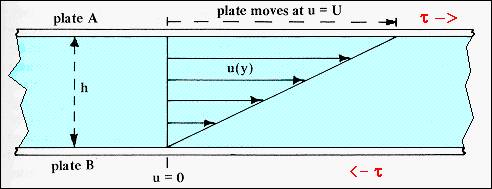Mechanics ViscosityViscosity is a property of a fluid that determines its flow behavior. The higher the viscosity the less easily the fluid can flow. Air has a low viscosity compared to water, and water a low viscosity compared to an automotive gear oil. The diagram shows a fluid between two solid plates. Plate B is fixed and plate A is moving with respect to it with a velocity, U. In the continuum limit, the fluid in contact with each plate moves at the same speed as the plate (a no slip condition). Between the two plates the fluid velocity increases from zero at plate B to U at plate A. The diagram shows a particular case for which the fluid velocity increases linearly with distance y from the plate: u(y) = U(y/h), where h is the separation of the two parallel plates. A shear stress, t = (F/A) must be applied to the system to keep the top plate moving at a constant speed U. This stress is related to the viscosity, m, of the fluid and the velocity gradient or strain rate (U/h): t = m (U/h). A fluid that behaves in this way is known as a Newtonian fluid and the expression as Newton's law of shear. For a Newtonian fluid the viscosity is isotropic. In SI units the viscosity is expressed in N.s/m2 or Poise.From: Wegener, "What Makes Airplanes Fly?" Springer-Verlag (1991)